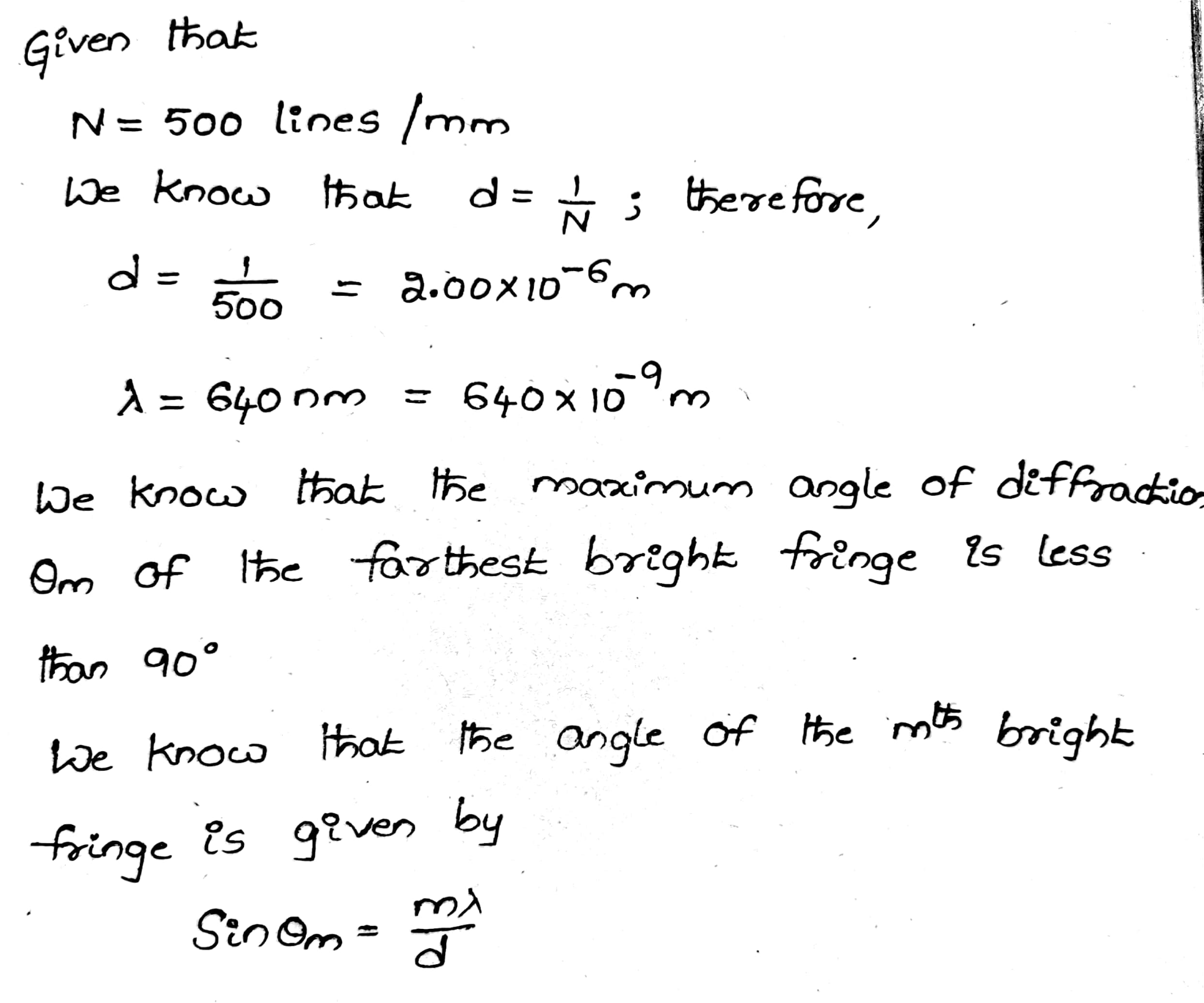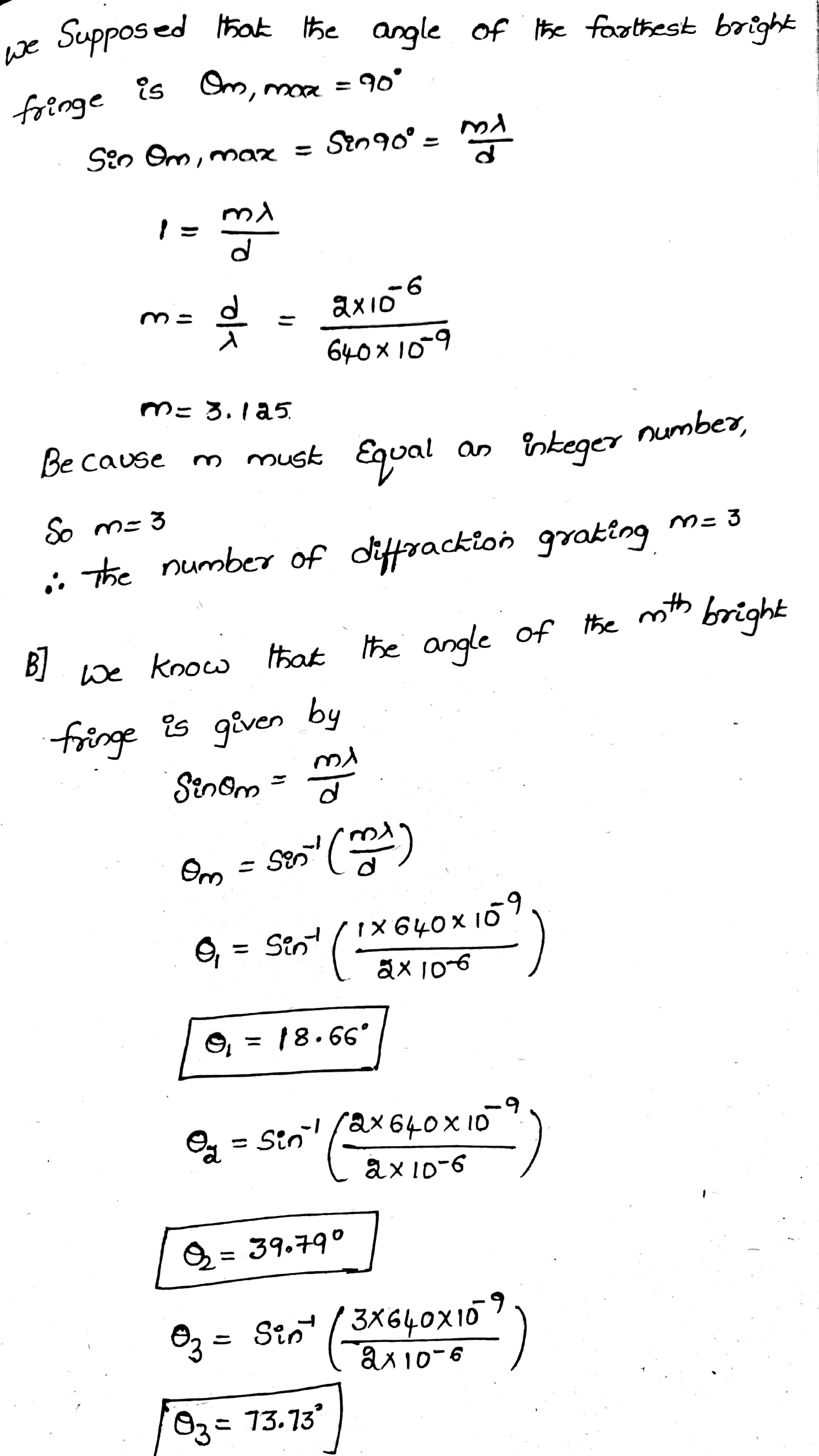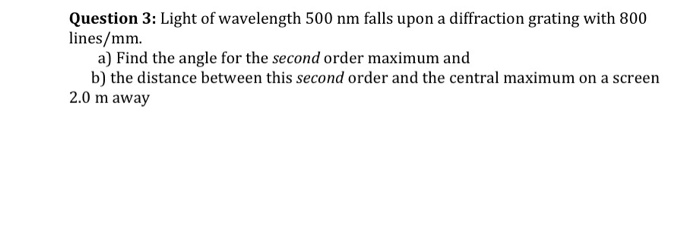Question

A 500 lines per mm diffraction grating is illuminated by light of wavelength 640 nm .

For the steps and strategies involved in solving a similar problem, you may view a Video Tutor Solution.

Part A

What is the maximum diffraction order seen? Express your answer as an integer.

Part B

What is the angle of each diffraction order starting from zero diffraction order to the maximum visible diffraction order?#### Earn Coins

Coins can be redeemed for fabulous gifts.

Similar Homework Help Questions
• ### A 500 lines per mm diffraction grating is illuminated by light of wavelength 560 nm ....

A 500 lines per mm diffraction grating is illuminated by light of wavelength 560 nm . Part A What is the maximum diffraction order seen Part B: What is the angle of each diffraction order starting from zero diffraction order to the maximum visible diffraction order? Enter your answers in degrees in ascending order separated by commas.

• ### A diffraction grating with 750 slits per mm is illuminated by light which gives a first-order...

A diffraction grating with 750 slits per mm is illuminated by light which gives a first-order diffraction angle of 34.0°. For the steps and strategies involved in solving a similar problem, you may view a Video Tutor Solution.    Part A What is the wavelength of the light?

• ### A 500 lines per mm diffraction grating is illuminated by light of wavelength 510...continues

A 500 lines per mm diffraction grating is illuminated by light of wavelength 510 nm. What is the angle of each diffraction order?

• ### A diffraction grating with 600 lines/mm is illuminated with light of wavelength 510 nm. A very...

A diffraction grating with 600 lines/mm is illuminated with light of wavelength 510 nm. A very wide viewing screen is 4.2 m behind the grating. Part A What is the distance between the two m = 1 bright fringes? Express your answer with the appropriate units. Δy = SubmitMy AnswersGive Up Part B How many bright fringes can be seen on the screen? N = SubmitMy AnswersGive Up

• ### A 480 lines/mm diffraction grating is illuminated by light of wavelength 510 nm . How many...

A 480 lines/mm diffraction grating is illuminated by light of wavelength 510 nm . How many bright fringes are seen on a 4.0-m-wide screen located 2.1 m behind the grating?

• ### Question 3: Light of wavelength 500 nm falls upon a diffraction grating with 800 lines/mm. a)...Question 3: Light of wavelength 500 nm falls upon a diffraction grating with 800 lines/mm. a) Find the angle for the second order maximum and b) the distance between this second order and the central maximum on a screen 2.0 m away

• ### A 500 line/mm diffraction grating is illuminated by light of wavelength 510nm. how many diffraction orders...

A 500 line/mm diffraction grating is illuminated by light of wavelength 510nm. how many diffraction orders are seen, and what is the angle of each?

• ### A 500 line/mm diffraction grating is illuminated by light of wavelength 510 nm. How many bright...

A 500 line/mm diffraction grating is illuminated by light of wavelength 510 nm. How many bright fringes are seen on a 2.0-m-wide screen located 2.0 m behind thegrating?

• ### A diffraction grating has 300 lines per mm. If light of wavelength 630 nm is sent...

A diffraction grating has 300 lines per mm. If light of wavelength 630 nm is sent through this grating, what is the highest order maximum that will appear? 2 5.3 5 8 6

• ### A 500 line/mm diffraction grating is illuminated by light of wavelength 510m

A 500 line/mm diffraction grating is illuminated by light of wavelength 510m. How many bright fringes are seen on a 2.0-m-wide screen located behind the grating?